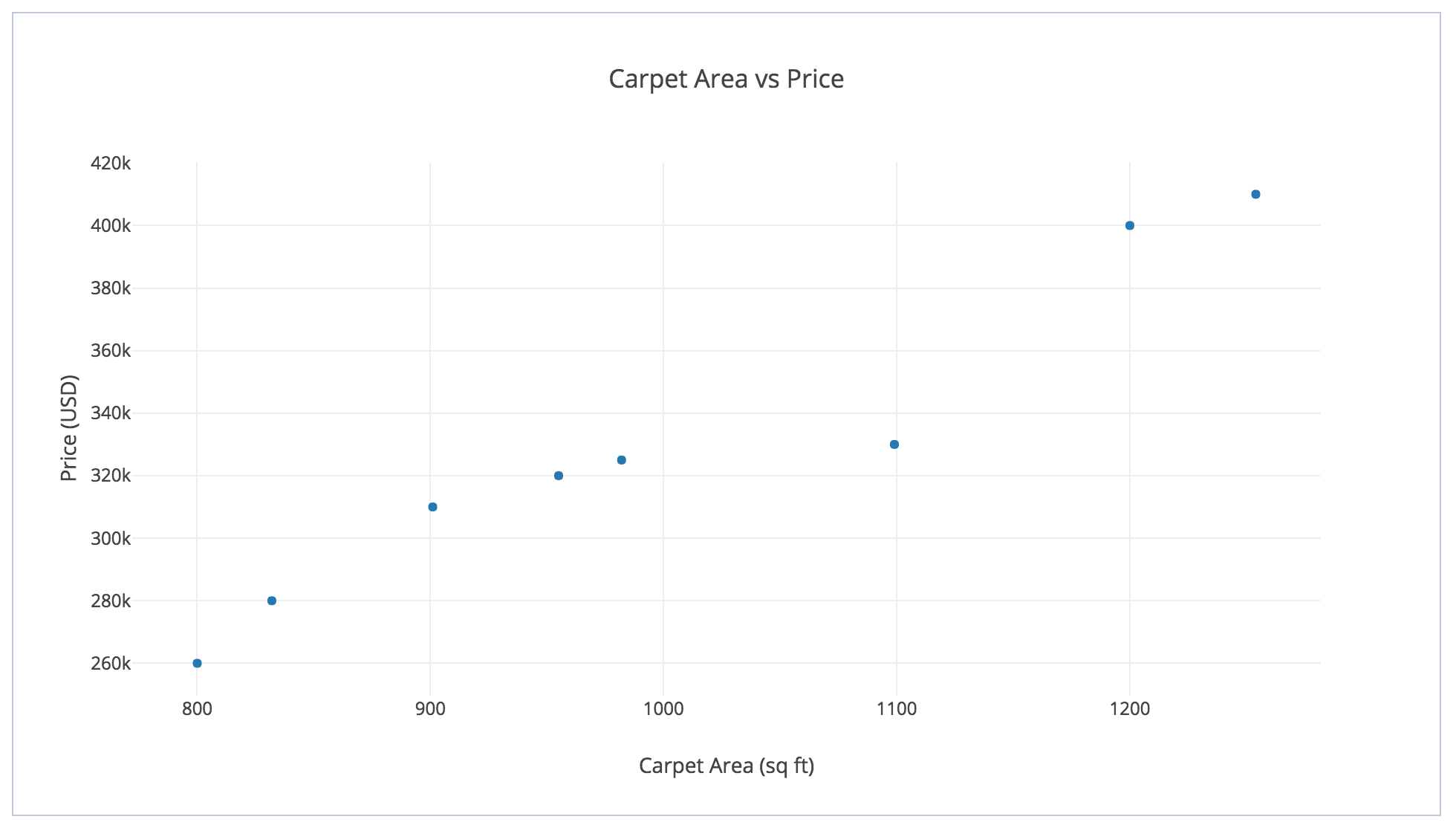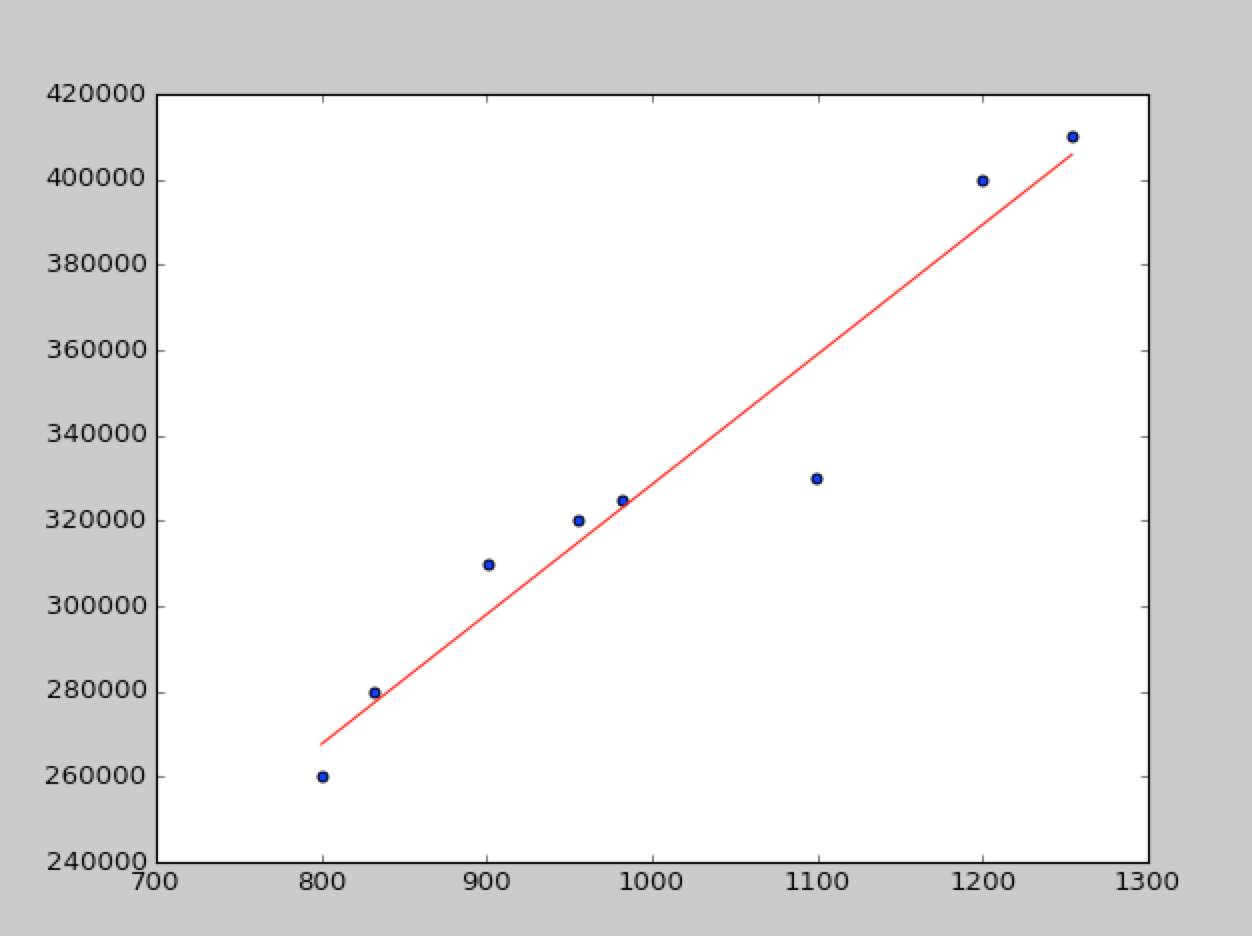# Linear Regression in Machine Learning

0
1153Often in real life, we face problems where we need to predict (or estimate) something given some information about that thing. As a simple example, when we purchase a house, we try to estimate the price of the house looking at various factors. Some of the factors include:

• Carpet area of the house in square feet.
• Number of rooms in the house.
• Type of locality nearby.
• Availability of other amenities like public transport, quality of roads, etc.

So basically, we use these parameters to estimate the price of the house. The price here is a positive number. For instance, it could be \$400,000.

Such problems in which we estimate a real value based on some input parameters are termed as regression problems. Basically, given our past knowledge of prices, we try to create a model of various parameters so as to estimate the price of a new house. Mathematically, it can be written as:

Estimated value = f(X)

Where X is the parameter vector that contains various values as mentioned above (area, number of rooms, etc) and f is the model function that maps X to an estimated value that reasonably well predicts the actual value.

For simplicity, let us assume that X is a 1 x 1 vector that contains exactly 1 real number – the Carpet area of the house in square feet. So X could look something like: X =  which signifies that the Carpet area is 1,200 square feet.

Take a look at some of the housing prices in certain areas of a particular City:

 Carpet area (Square feet) Price (USD) 800 260,000 832 280,000 901 310,000 955 320,000 982 325,000 1099 330,000 1200 400,000 1254 410,000

When plotted on the graph, it looks something like this:As can be seen from the graph above, the points are approximately aligned in a straight line. Basically, we can approximately draw a line that relates the price of the house with the Carpet area.

The line could look something like this:Now, the question arises – how to pick this line? Basically, can we find out a way by which we can decide on the equation of this line?

This is the question that Linear Regression tries to answer. Let us assume that the equation of this line is y = a0 * x + a1 where x is the carpet area of the house and a0 and a1 are constants that we need to find out. We need to find a way by which we can find the best values of a0 and a1.

The way we do it is that we try to optimize the error that happens in the predictions. For instance, if for a certain carpet area x the actual price is y. Our algorithm will predict it to be y = a0 * x + a1. The error in measurement is (y – [a0 * x + a1]). The error maybe positive or negative. However, we want to make sure that we count only the positive error. A way to achieve this is to rather have the error metric as the square of the above error: (y – [a0 * x + a1])2. Summing this up for all points, we have:

E(a0, a1) = (y1 – [a0 * x1 + a1])2 + (y2 – [a0 * x2 + a1])2 + .. + (yn – [a0 * xn + a1])n

In Linear Regression, we try to find out the values of a0 and a1 which minimizes this error function. This error function is often called as cost function.

As can be seen that the error function above is a quadratic function of a0 and a1 and so, the above problem is simply an optimization problem which could be solved by various techniques. Some of the techniques are:

• Partial Differentiation

Discussion of these techniques is beyond the scope of this blog. However, this is where Python comes to the rescue. Python provides a powerful library called Scikit Learn which abstracts out this optimization work for you. Let us take a look at a sample code:

```# import the necessary packages
import matplotlib.pyplot as plt
from sklearn import linear_model

# create the sample dataset
X = [, , , , , , , ]
Y = [260000, 280000, 310000, 320000, 325000, 330000, 400000, 410000]

# create the model
regression_models = linear_model.LinearRegression()
regression_models.fit(X, Y)

# get the values of parameters from the model
a0 = regression_models.coef_
a1 = regression_models.intercept_

# get the predictions
predictions = []
for i in X:
predictions.append(a0 * i + a1)

# plot the graph
plt.scatter(X, Y)
plt.plot(X, predictions, 'r')
plt.show()```

On running the above code, we will get a graph that looks something like this:Let us now understand the code part-by-part

```# import the necessary packages
import matplotlib.pyplot as plt
from sklearn import linear_model```
```# import the necessary packages
import matplotlib.pyplot as plt
from sklearn import linear_model```

Here, we are importing the matplotlib library which is used for plotting the graph and also the sklearn packages to be used for performing linear regression.

```# create the sample dataset
X = [, , , , , , , ]
Y = [260000, 280000, 310000, 320000, 325000, 330000, 400000, 410000]```

Here, we just created the training dataset.

```# create the model
regression_models = linear_model.LinearRegression()
regression_models.fit(X, Y)```

Here, we are trying to fit a model on the training dataset.

```# get the values of parameters from the model
a0 = regression_models.coef_
a1 = regression_models.intercept_```

Model fitting is done. Here, we are trying to obtain the model parameters.

```# get the predictions
predictions = []
for i in X:
predictions.append(a0 * i + a1)```

Here, we have obtained the predictions from the model.

```# plot the graph
plt.scatter(X, Y)
plt.plot(X, predictions, 'r')
plt.show()```

Finally, we plot the graph of the points as well as the obtained straight line.

As can be seen from the image above, this simple code of Linear Regression gives us a reasonably good straight line. The line seems to generalize the price of a house based on the Carpet area. Using this line, we can predict the prices of unknown Carpet area houses. This will help us attach a price to a house which we plan to purchase.

Linear Regression is a powerful tool and it’s like the “Hello World!” of Machine Learning. You should spend more time in understanding it thoroughly to develop a deeper understanding of the ML domain.